# Quickly Typing Dates into Cells

In this article we will learn about how we can enter the quickly into cells in Microsoft Excel 2013.

Typing a large amount of data into cells can be exhausting especially if it includes a series of dates.

If you have only one date in a cell and you want to present it as a date series use the Date formula along with Today formula in Microsoft Excel 2010 and 2013.

Date: -Returns the number that represent the date in Microsoft Excel date-time code.

Syntax of “Date” function:            =DATE(Year,Month,Day)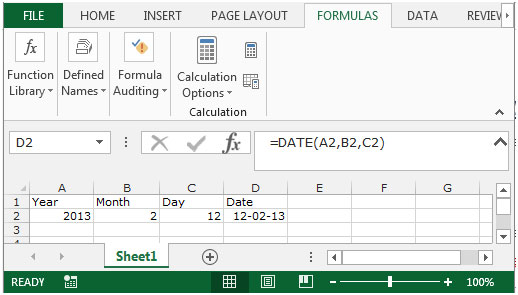Today: - Returns the current date formatted as a date.

Syntax of “Today” function:         =TODAY()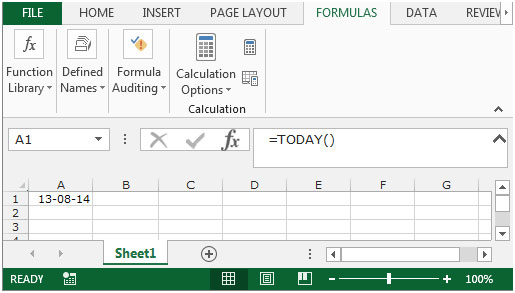Let’s take an example and understand, we have days number in column A. We want to convert that day number into Date including with current month and year.

Date function along with”Today” function

• Select the cell B2 and type the day (serial number only)into range A2:A6.
• Add the formula to insert the month and year in cell B2.
• =DATE(YEAR(TODAY()),MONTH(TODAY()),A2), press enter.
• The function will return the date with current month and year.
• To return the date for the all rest of days number copy the same formula by pressing the key Ctrl+C and paste it in the range B3:B6 by pressing the key Ctrl+V.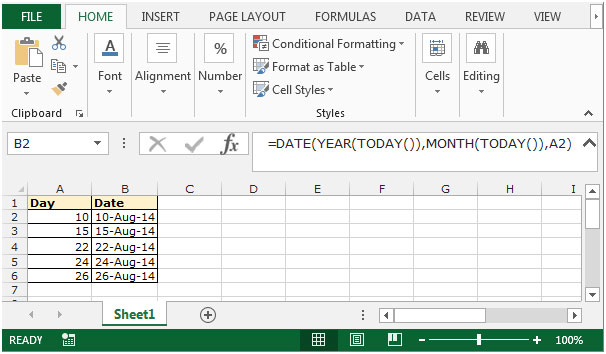To convert the number into date format we use the “Date value” function along with “Left”, “Mid”, and “Right” function.

LEFT: Returns the first character(s) in a text string based on the number of characters specified.

Syntax of “LEFT” function:            =LEFT (text,[num_chars])

Example:Cell A1contains the text “Broncho Billy Anderson”

=LEFT (A1, 7), function will return “Broncho”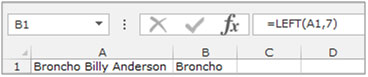RIGHT:Return the last character(s) in a text string based on the number of characters specified.

Syntax of “RIGHT” function:        =RIGHT (text, [num_chars])

Example:Cell A1contains the text “Broncho Billy Anderson”

=RIGHT (A1, 8), function will return “Anderson”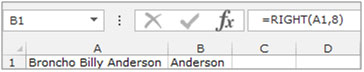MID:Return a specific number of character(s) from a text string, starting at the position specified based on the number of characters specified.

Syntax of “MID” function:=MID (text,start_num,num_chars)

Example:Cell A2 contains the text “Broncho Billy Anderson”

=MID (A1, 8, 7), function will return “Billy”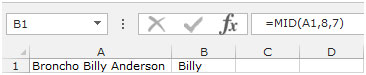Date Value:Converts a date in the form of text to a number that represents the date in Microsoft Excel.

Syntax of “DATEVALUE” function:           =DATEVALUE (date_text)

Date Function Along with “Left”, “Mid”, “Right”

We have without formatted date in range A2:A6. We want to format the numbers in date format.

• Select the cell B2 and type a full number.
• =DATEVALUE(LEFT(A3,2)&”/”&MID(A3,3,2)&”/”&RIGHT(A3,2))
• And press enter.
• The function will return the date with current month and year.
• To return the date for the all rest of day’s number copy the same formula by pressing the key Ctrl+C and paste it in the range B3:B6 by pressing the key Ctrl+V.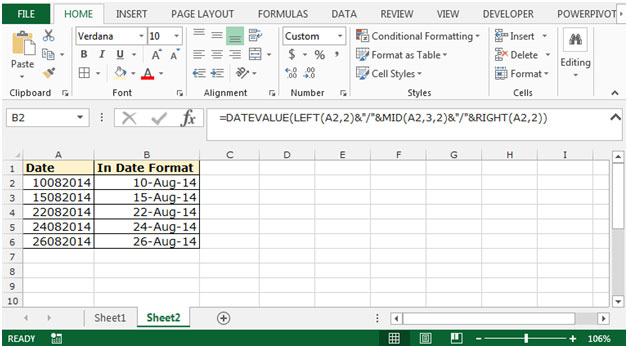This way we can quickly type dates into cell in Microsoft Excel 2010 and 2013.

## Users are saying about us...

1.I want to know how to show a number, twice its value. For example showing 2 boxes of data into one. So I would have a 14, and I would want to show as a 28.

Terms and Conditions of use

The applications/code on this site are distributed as is and without warranties or liability. In no event shall the owner of the copyrights, or the authors of the applications/code be liable for any loss of profit, any problems or any damage resulting from the use or evaluation of the applications/code.